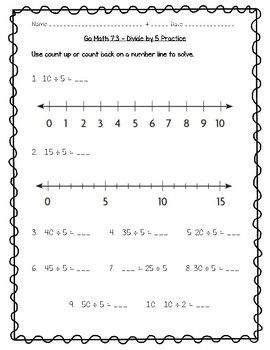# Go Math Practice - 3rd Grade Chapter 7 - Division Facts and StrategiesSubject
Resource Type
File Type
PDF (2 MB|26 pages)
Standards
\$5.00
Also included in:
1. What's IncludedIncluded in this pack are worksheets on all the lessons in the third grade Go Math series, a midchapter checkpoint practice sheet, and an end of the chapter checkpoint practice sheet for each chapter.These can be used as a quiz, formative assessment, homework, or just extra practice!A
\$60.00
\$50.00
Save \$10.00
• Product Description
• Standards
What's Included

Included in this pack are 13 worksheets on all the lessons in the third grade Go Math book for chapter 7, a midchapter checkpoint practice sheet, and an end of the chapter checkpoint practice sheet.

These can be used as a quiz, formative assessment, homework, or just extra practice!

Answer keys are included for each worksheet.

Lesson 1: Divide by 2
Lesson 2: Divide by 10
Lesson 3: Divide by 5
Lesson 4: Divide by 3
Lesson 5: Divide by 4
Lesson 6: Divide by 6
Mid-Chapter Checkpoint (lessons 1-6)
Lesson 7: Divide by 7
Lesson 8: Divide by 8
Lesson 9: Divide by 9
Lesson 10: Problem Solving • Two-Step Problems
Lesson 11: Investigate • Order of Operations
End of Chapter Checkpoint (lessons 7-11)

3.OA.A.3, 3.OA.C.7, 3.OA.D.8,

Sample 7.1 with this freebie

Chapter 1 - Addition and Subtraction Within 1,000
Chapter 2 - Represent and Interpret Data
Chapter 3 - Understand Multiplication
Chapter 4 - Multiplication Facts and Strategies
Chapter 5 - Use Multiplication Facts
Chapter 6 - Understand Division
Chapter 8 - Understand Fractions
Chapter 9 - Compare Fractions
Chapter 10 - Time, Length, Liquid Volume, & Mass
Chapter 11 - Perimeter and Area
Chapter 12 - Two-Dimensional Shapes
Solve two-step word problems using the four operations. Represent these problems using equations with a letter standing for the unknown quantity. Assess the reasonableness of answers using mental computation and estimation strategies including rounding.
Fluently multiply and divide within 100, using strategies such as the relationship between multiplication and division (e.g., knowing that 8 × 5 = 40, one knows 40 ÷ 5 = 8) or properties of operations. By the end of Grade 3, know from memory all products of two one-digit numbers.
Use multiplication and division within 100 to solve word problems in situations involving equal groups, arrays, and measurement quantities, e.g., by using drawings and equations with a symbol for the unknown number to represent the problem.
Total Pages
26 pages